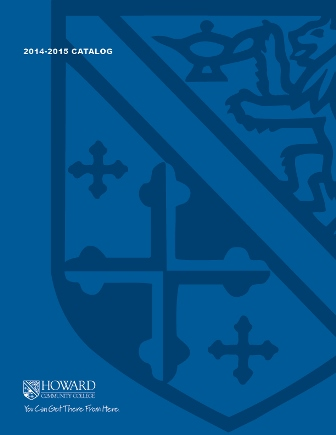2017-2018 College Catalog# MATH 128 Concepts of Mathematics II

This course is the second course in a sequence intended primarily for students in the elementary and early childhood education programs. Topics include probability, metric and non-metric geometry, dimensional analysis, congruence and similarity, and coordinate and transformational geometry. Problem-solving techniques are emphasized including the appropriate use of calculators and computers. This is a general education core course for designated education programs only.

4

### Prerequisite

C or better in MATH 070 or appropriate score on the mathematics placement test

4 hours weekly

### Course Objectives

1. 1. Communicate mathematical concepts about relationships among points, lines, and planes,
the image of a geometric reflection, and the characteristics of a variety of polygons including
symmetry using appropriate symbols, notation, and vocabulary
2. 2. Solve application problems involving multi-stage experiments with tree diagrams, geometric
probabilities, similar triangles, and other similar figures, translations, and rotations, threedimensional
geometry, and application problems involving measurement, mass, and
temperature.
3. 3. Identify and implement appropriate technologies to efficiently complete tasks that involve the
solving of cross-discipline, mathematically appropriate problems and creating new works to
communicate the processes used and solution.
4. 4. Analyze, evaluate, justify, and interpret the reasonableness of solutions, particularly in the
area of geometric conjectures.

### Course Objectives

1. Communicate mathematical concepts about relationships among points, lines, and planes,
the image of a geometric reflection, and the characteristics of a variety of polygons including
symmetry using appropriate symbols, notation, and vocabulary

2. Solve application problems involving multi-stage experiments with tree diagrams, geometric
probabilities, similar triangles, and other similar figures, translations, and rotations, threedimensional
geometry, and application problems involving measurement, mass, and
temperature.

3. Identify and implement appropriate technologies to efficiently complete tasks that involve the
solving of cross-discipline, mathematically appropriate problems and creating new works to
communicate the processes used and solution.

4. Analyze, evaluate, justify, and interpret the reasonableness of solutions, particularly in the
area of geometric conjectures.